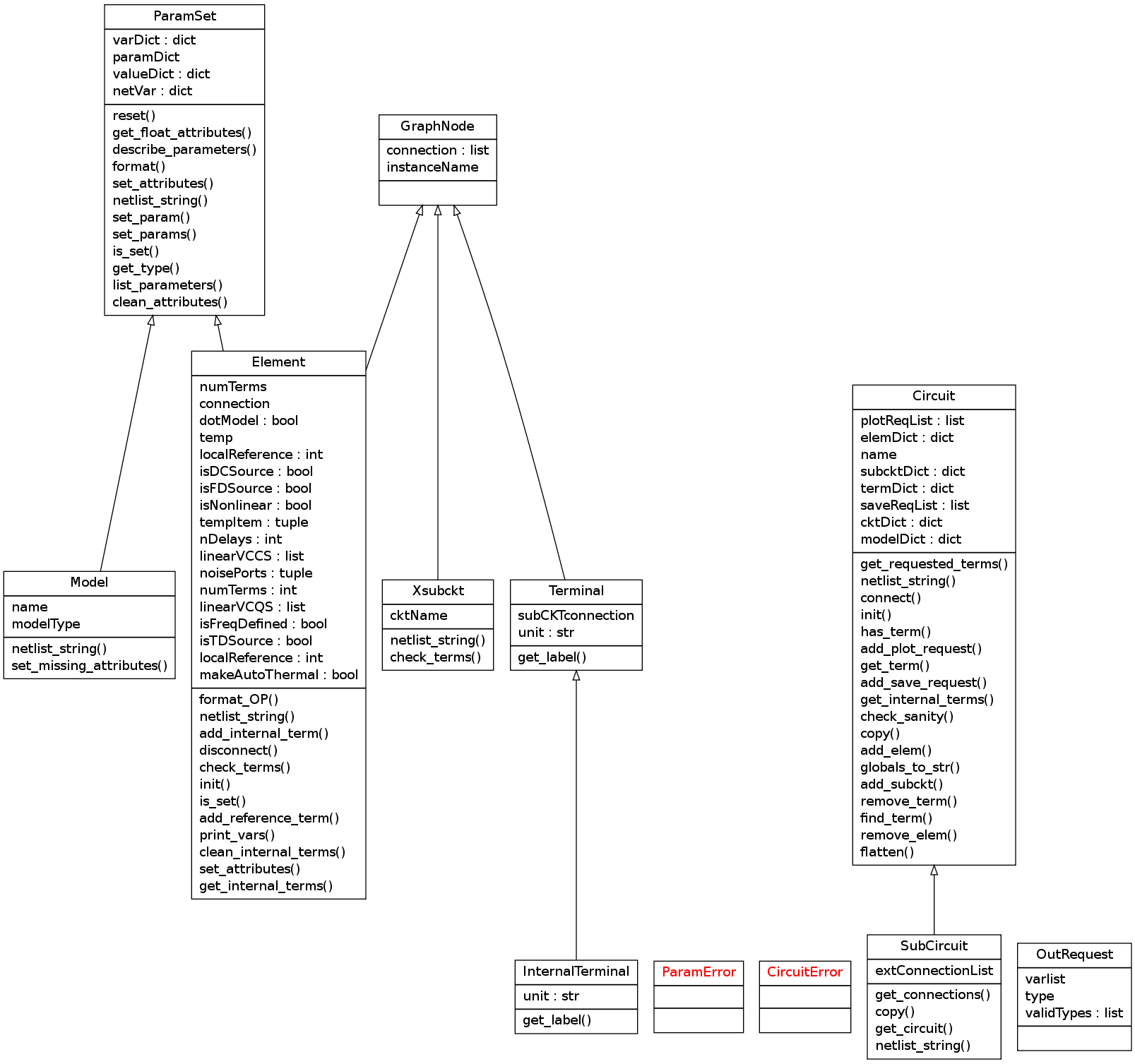# Internal Circuit Representation Reference¶

This document covers only how a circuit is represented after a netlist is parsed. This representation attempts to be independent of how the circuit is analyzed. Any specific analysis approach should be implemented separately in the analysis package.

## UML Class Diagram¶All device model classes are derived from the Element class.

## Modules and classes¶

### circuit – Classes for internal circuit representation¶

#### Example of how to use this module¶

The following example was originally taken from analyses/nodal.py. The objective of this code is to assign row/column numbers to all non-reference nodes and -1 to all reference nodes in a Circuit instance (ckt):

```# make a list of all non-reference terminals in circuit
ckt.nD_termList = ckt.termDict.values() + ckt.get_internal_terms()
# get reference node (if any)
if ckt.has_term('gnd'):
ckt.nD_ref = ckt.get_term('gnd')
# remove ground node from terminal list
ckt.nD_termList.remove(ckt.nD_ref)
# remove ground node from terminal list
ckt.nD_termList.remove(ckt.nD_ref)
# For reference nodes assign -1
ckt.nD_ref.nD_namRC = -1
# Make a list of all elements
ckt.nD_elemList = ckt.elemDict.values()
# Set RC number of reference terminals to -1
for elem in ckt.nD_elemList:
if elem.localReference:
elem.connection[elem.localReference].nD_namRC = -1
# Assign a number (starting from 0) to all nodes.
for i, term in enumerate(ckt.nD_termList):
term.nD_namRC = i
```

The following example shows how to create and add devices from the point of view of a parser:

```import circuit as cir
from devices import devClass

# Create circuit:
mainckt = cir.Circuit()

# Add elements with private model

# creates element type 'ind'
dev = devClass['ind']('Lchoke')

# override 'l' parameter value (otherwise default value is used)
dev.set_param('l', 1e-9)

# connect to terminals 'vdd' and and 'drain', automatically created
mainckt.connect(dev, ['vdd', 'drain'])

# add element with shared (public) model: model 'my_nmos' is created
# if it is not already in circuit
m1 = devClass['mosacm']('mos1n')

# To later retrieve model
model = cir.get_model('my_nmos')

# Subcircuits: create and add subcircuit instance
x1 = cir.Xsubckt('x1', 'LM741')
# Connect subcircuit instance with cir.connect()

# Subcircuit definition: tl2 are the external connections
tl2 = ['vdd', 'vee', 'in', 'out']
amp1 = cir.SubCircuit('LM741', tl2)
# now you can treat amp1 as a regular circuit instance
dev = devClass['ind']('L2')
dev.override = [('l', 2e-9)]
amp1.connect(dev, ['in', 'n1'])
```